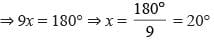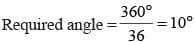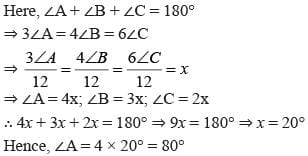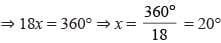Olympiad Test : Geometry - 2

# Olympiad Test : Geometry - 2

Test Description

## 20 Questions MCQ Test Maths Olympiad Class 6 | Olympiad Test : Geometry - 2

Olympiad Test : Geometry - 2 for Class 6 2022 is part of Maths Olympiad Class 6 preparation. The Olympiad Test : Geometry - 2 questions and answers have been prepared according to the Class 6 exam syllabus.The Olympiad Test : Geometry - 2 MCQs are made for Class 6 2022 Exam. Find important definitions, questions, notes, meanings, examples, exercises, MCQs and online tests for Olympiad Test : Geometry - 2 below.
Solutions of Olympiad Test : Geometry - 2 questions in English are available as part of our Maths Olympiad Class 6 for Class 6 & Olympiad Test : Geometry - 2 solutions in Hindi for Maths Olympiad Class 6 course. Download more important topics, notes, lectures and mock test series for Class 6 Exam by signing up for free. Attempt Olympiad Test : Geometry - 2 | 20 questions in 40 minutes | Mock test for Class 6 preparation | Free important questions MCQ to study Maths Olympiad Class 6 for Class 6 Exam | Download free PDF with solutions
 1 Crore+ students have signed up on EduRev. Have you?
Olympiad Test : Geometry - 2 - Question 1

### The sum of all angles of a quadrilateral is

Olympiad Test : Geometry - 2 - Question 2

### A quadrilateral having one and only one pair of parallel sides is

Olympiad Test : Geometry - 2 - Question 3

### How many parts does a triangle have?

Olympiad Test : Geometry - 2 - Question 4

The angle of a triangle are in the ratio 2 : 3 : 4. The smallest angle is

Detailed Solution for Olympiad Test : Geometry - 2 - Question 4

Here,
2x + 3x + 4x = 180°Smallest angle = 2x = 2 × 20° = 40°

Olympiad Test : Geometry - 2 - Question 5

One of the base angle of an isosceles triangle is 65°. The vertical angle is

Detailed Solution for Olympiad Test : Geometry - 2 - Question 5

Vertical angle = 180° – (65° + 65°)
= 180° – 130° = 50°

Olympiad Test : Geometry - 2 - Question 6

Each angle of an equilateral triangle is

Olympiad Test : Geometry - 2 - Question 7

An angle measuring 360° is

Olympiad Test : Geometry - 2 - Question 8

The measure of a straight angle is

Olympiad Test : Geometry - 2 - Question 9

An angle measuring 205° is

Olympiad Test : Geometry - 2 - Question 10

If there are 36 spokes in a bicycle wheel then the angle between a pair of adjacent spokes is

Detailed Solution for Olympiad Test : Geometry - 2 - Question 10Olympiad Test : Geometry - 2 - Question 11

The minimum number of points of intersection of three lines in a plane is

Olympiad Test : Geometry - 2 - Question 12

Two planes intersect

Olympiad Test : Geometry - 2 - Question 13

One of the acute angle of a right triangle is 55°. What is the other acute angle?

Detailed Solution for Olympiad Test : Geometry - 2 - Question 13

Other angle = 180° – (90° + 55°)
= 180° – 145° = 35°

Olympiad Test : Geometry - 2 - Question 14

In a ∆ABC, 3∠A = 4∠B = 6∠C. What is the measure of largest angle?

Detailed Solution for Olympiad Test : Geometry - 2 - Question 14Olympiad Test : Geometry - 2 - Question 15

A triangle having sides of different length is called

Olympiad Test : Geometry - 2 - Question 16

The angles of a quadrilateral are in the ratio 3 : 4 : 5 : 6. What is the largest angle

Detailed Solution for Olympiad Test : Geometry - 2 - Question 16

3x + 4x + 5x + 6x = 360°∴ Largest angle = 6 × 20° = 120°

Olympiad Test : Geometry - 2 - Question 17

A quadrilateral having two pairs of equal adjacent sides but unequal opposite sides is

Olympiad Test : Geometry - 2 - Question 18

A cone has how many vertex?

Olympiad Test : Geometry - 2 - Question 19

A brick is an example of

Olympiad Test : Geometry - 2 - Question 20

How many vertex a cylinder has?

## Maths Olympiad Class 6

43 tests
 Use Code STAYHOME200 and get INR 200 additional OFF Use Coupon Code
Information about Olympiad Test : Geometry - 2 Page
In this test you can find the Exam questions for Olympiad Test : Geometry - 2 solved & explained in the simplest way possible. Besides giving Questions and answers for Olympiad Test : Geometry - 2, EduRev gives you an ample number of Online tests for practice

43 tests